GeeksforGeeks App
Open AppBrowser
Continue

# Java Program to Handle Divide By Zero and Multiple Exceptions

Exceptions These are the events that occur due to the programmer error or machine error which causes a disturbance in the normal flow of execution of the program.

Handling Multiple exceptions: There are two methods to handle multiple exceptions in java.

1. Using a Single try-catch block try statement allows you to define a block of code to be tested for errors, and we can give exception objects to the catch blow because this all the exceptions inherited by the Exception class.
2. The second method is to create individual catch blocks for the different exception handler.

Hierarchy of the exceptions: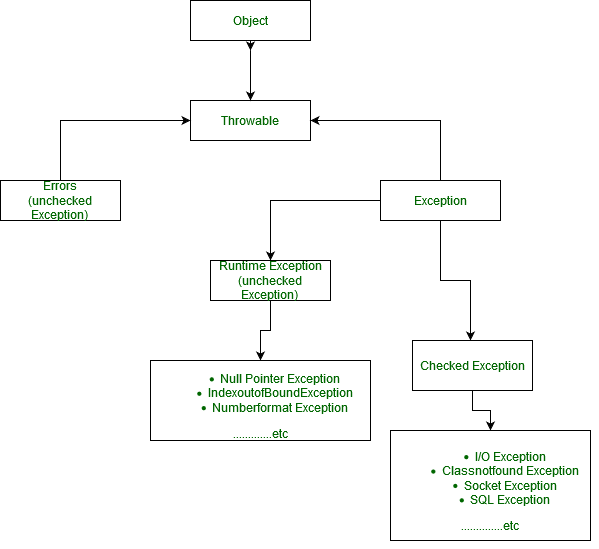Divide by zero: This Program throw Arithmetic exception because of due any number divide by 0 is undefined in Mathematics.

## Java

 `// Java Program to Handle Divide By Zero Exception``import` `java.io.*;``class` `GFG {``    ``public` `static` `void` `main(String[] args)``    ``{``        ``int` `a = ``6``;``        ``int` `b = ``0``;``        ``System.out.print(a / b);``        ``// this line Throw  ArithmeticException: / by zero``    ``}``}`

Output: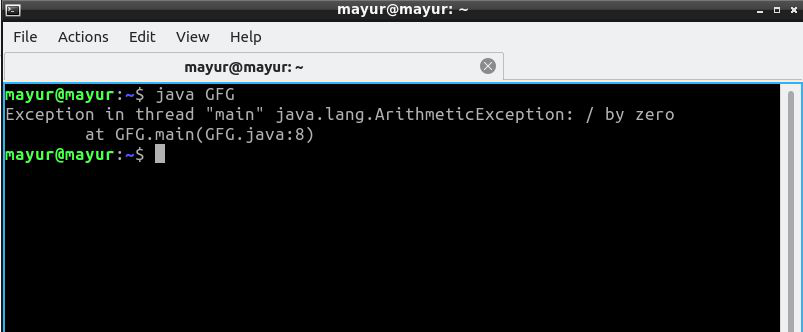Handling of Divide by zero exception: Using try-Catch Block

## Java

 `// Java Program to Handle Divide By Zero Exception``import` `java.io.*;``class` `GFG {``    ``public` `static` `void` `main(String[] args)``    ``{``        ``int` `a = ``5``;``        ``int` `b = ``0``;``        ``try` `{``            ``System.out.println(a / b); ``// throw Exception``        ``}``        ``catch` `(ArithmeticException e) {``            ``// Exception handler``            ``System.out.println(``                ``"Divided by zero operation cannot possible"``);``        ``}``    ``}``}`

Output: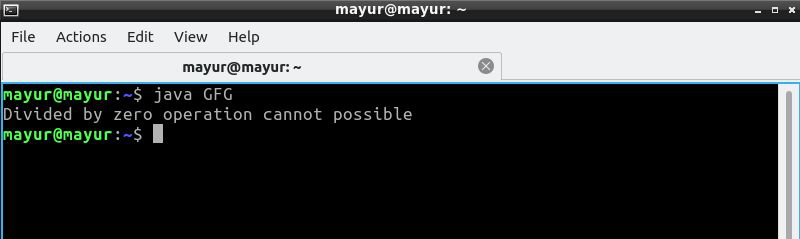Multiple Exceptions (ArithmeticException and IndexoutOfBound Exception)

1. Combination of two Exception using the | operator is allowed in Java.
2. As soon as the first exception occurs it gets thrown at catch block.
3. Check of expression is done by precedence compiler check rule from right to left of the expression.

## Java

 `// Java Program to Handle multiple exception``import` `java.io.*;` `class` `GFG {``    ``public` `static` `void` `main(String[] args)``    ``{``        ``try` `{``            ``int` `number[] = ``new` `int``[``10``];``            ``number[``10``] = ``30` `/ ``0``;``        ``}``        ``catch` `(ArithmeticException e) {``            ``System.out.println(``                ``"Zero cannot divide any number"``);``        ``}``        ``catch` `(ArrayIndexOutOfBoundsException e) {``            ``System.out.println(``                ``"Index out of size of the array"``);``        ``}``    ``}``}`

Output: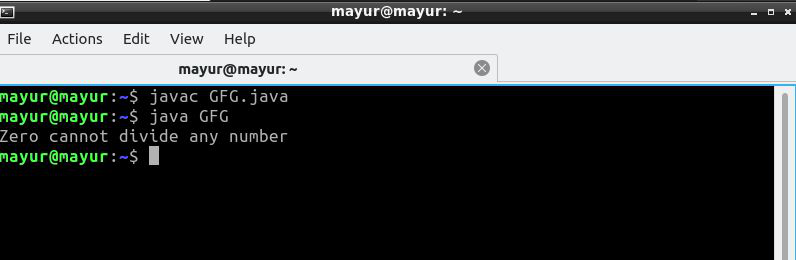Explanation: Here combination of ArrayIndexOutOfBounds and Arithmetic exception occur, but only Arithmetic exception is thrown, Why?

According to the precedence compiler check number=30/0 from right to left. That’s why 30/0 to throw ArithmeticException object and the handler of this exception executes Zero cannot divide any number.

Another Method of Multiple Exception: we can combine two Exception using the | operator and either one of them executes according to the exception occurs.

## Java

 `// Java Program to Handle multiple exception``import` `java.io.*;` `class` `GFG {``    ``public` `static` `void` `main(String[] args)``    ``{``        ``try` `{``            ``int` `number[] = ``new` `int``[``20``];``            ``number[``21``] = ``30` `/ ``9``;``            ``// this statement will throw``            ``// ArrayIndexOutOfBoundsException e``        ``}``        ``catch` `(ArrayIndexOutOfBoundsException``               ``| ArithmeticException e) {``            ``System.out.println(e.getMessage());``            ``// print the message``        ``}``    ``}``}`

Output: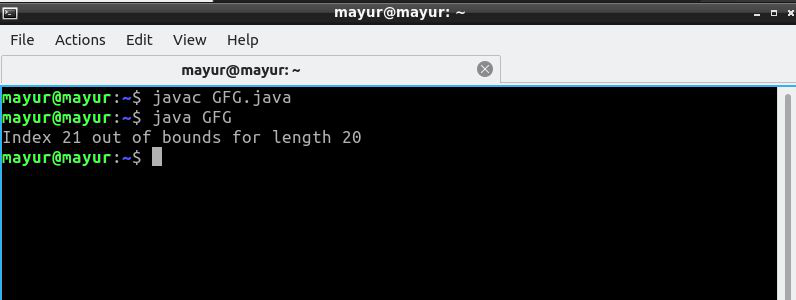My Personal Notes arrow_drop_up